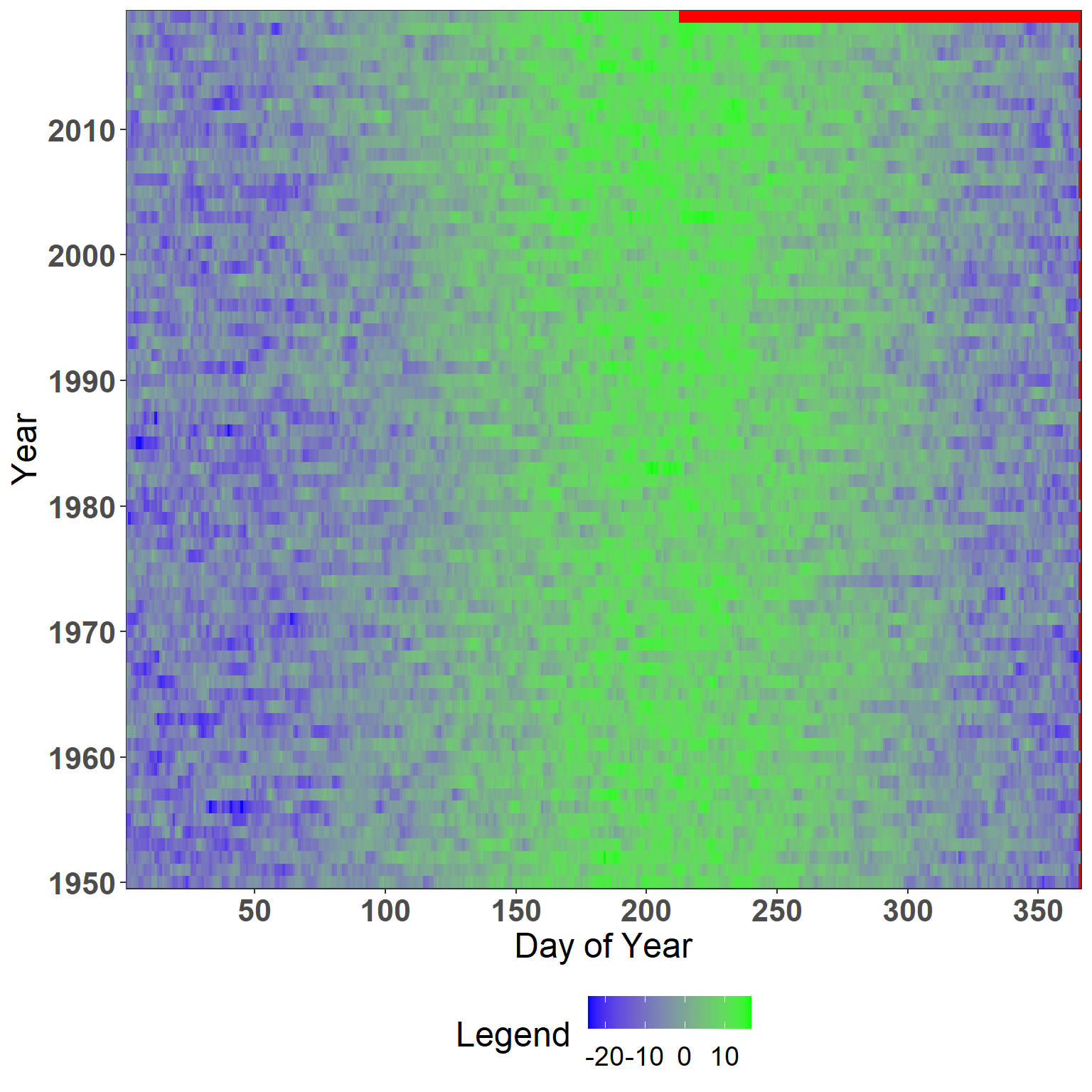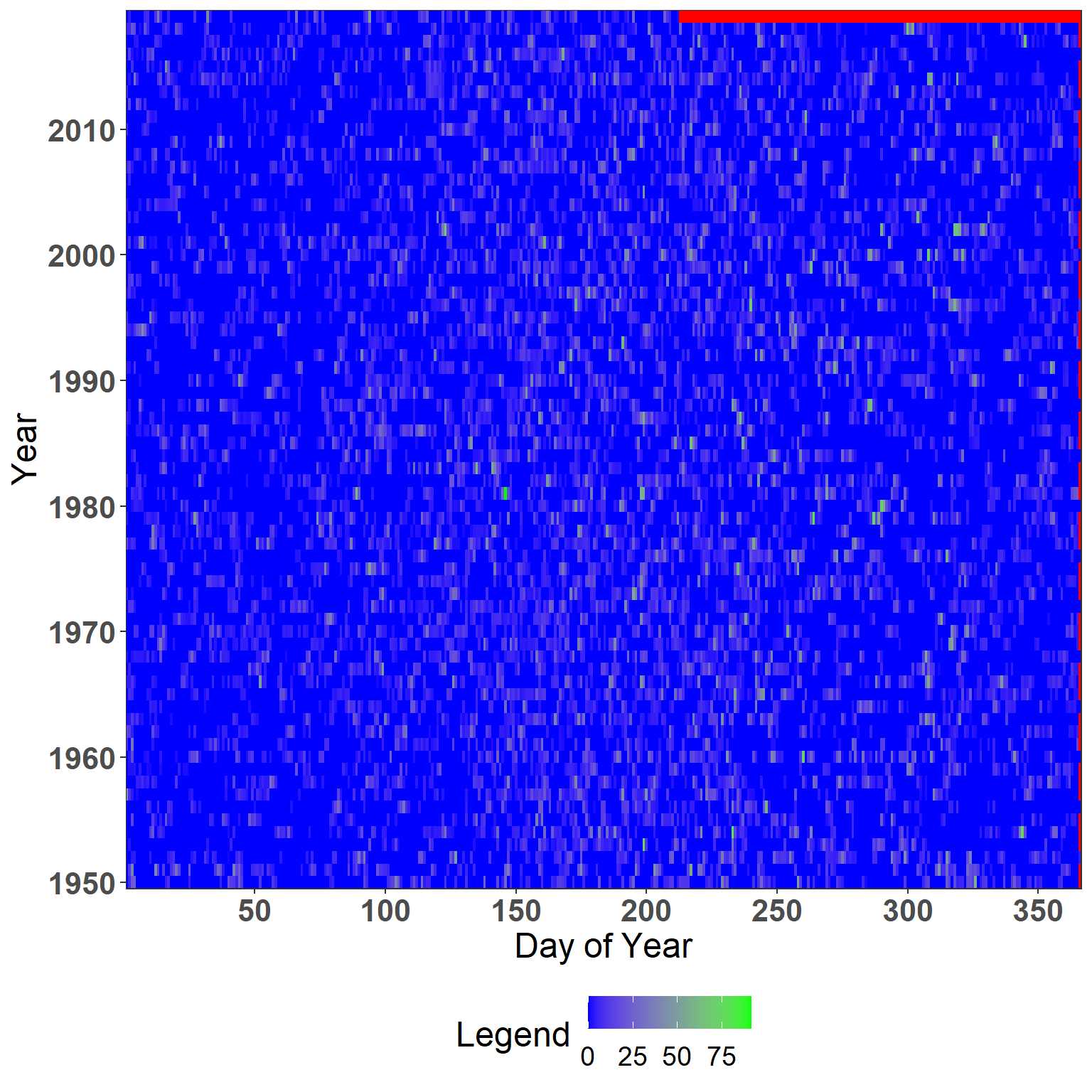# 1 Introduction

The dendroTools R package was developed in 2018 and is still being updated regularly and will continue to do so in the future. It provides dendroclimatological methods for analysing statistical relationships between tree rings and daily climate data. The most commonly used method is the Pearson correlation coefficient, but users can also use non-parametric correlations such as Kendall or Spearman correlations, and in the case of a multiproxy approach, linear regression can also be applied. Artificial neural networks (brnn) are also available for nonlinear analyses.

In this document I describe the basic principles behind the dendroTools R package and give some basic examples. All data included in the examples below is already included in the dendroTools R package. Please note that the examples presented here are less made computationally less intensive to accommodate the policy of CRAN. You are welcome to explore the full potential of my package by using the wide range of possible window widths.

# 2 Transformation and quick preview of daily data

One of the crucial step before using a dendroTools is to prepare climate data into a proper format. For daily_response() climate data must be in a format of 366 columns and n number of rows, which represent years, which are given as row names. A common format of daily data provided by many online sources is a table with two columns, where one column represents the date and the second is the value of the climate variable. To quickly transform such a format into a data frame with dimensions of 366 x n, dendroTools now offers the function data_transform(). The date can be in different formats, but it must be correctly speciﬁed with the argument date_format. For example, if the date is in the format “1988-01-30′′ (”year-month-day”), the argument date_format must be “ymd”.

When your data is in the proper format, the glimpse_daily_data() can be used for visual inspection of daily climate data. The main purpose here is to spot missing values and to evaluate the suitability of using specific daily data.

``````# Load the dendroTools and ggplot2 R packages
library(dendroTools)
library(ggplot2)

# 1 Load an example data (source: E-OBS)
data("swit272_daily_temperatures")
data("swit272_daily_precipitation")

# 2 Transform data into wide format
swit272_daily_temperatures <- data_transform(swit272_daily_temperatures, format = 'daily', date_format = 'ymd')
swit272_daily_precipitation <- data_transform(swit272_daily_precipitation, format = 'daily', date_format = 'ymd')

# 3 Glimpse daily data
glimpse_daily_data(env_data = swit272_daily_temperatures, na.color = "red") +
theme(legend.position = "bottom")``````Figure 1: Glimpse of daily temperautres for swit272 site.

``````glimpse_daily_data(env_data = swit272_daily_precipitation, na.color = "red") +
theme(legend.position = "bottom")``````Figure 2: Glimpse of daily precipitation for swit272 site.

# 3 The daily_response() in action

The daily_response() is the most commonly used function in the R package dendroTools, and works by sliding a moving window through the daily environmental (climate) data and computing statistical metrics using a tree-ring proxy. In dendroclimatology, such an analysis typically involves a site chronology that has been previously detrended, but a multiproxy approach involving multiple individual chronologies can also be used (see examples below). Possible metrics for the single-proxy approach include correlation coefficients, coefficient of determination (r-squared), and adjusted coefficient of determination (adjusted r-squared). In addition to linear regression, it is possible to use a nonlinear artificial neural network with a Bayesian regularisation training algorithm (brnn). In general, the user can use a fixed window or a progressive window to calculate the moving averages. To use a fixed window, choose its width by assigning an integer to the fixed_width argument. To use a so-called variable window, which includes many different windows, define the arguments lower_limit and upper_limit. In this case, all window widths between the lower and upper limits are taken into account. The window width is defined here as the number of days between the start and end days of a calculation. Thus, the window width represents a season of interest used in the calculations. All calculated statistical metrics (correlation coefficients, r-squared or adjusted r-squared) are stored in a matrix that is later used to interpret the results. Such interpretation usually involves identifying the optimal season in relation to tree-ring chronology or analysing temporal patterns of correlations between climate and growth. The so-called optimal season (also called optimal window or time window with the highest correlation value) is later extracted and used to evaluate the temporal stability correlations.

## 3.1 An example of analysing the relationship between Mean Vessel Area (MVA) tree-ring parameter and daily temperature data with fixed window approach

In this example, I analyse the relationship between the tree ring parameter Mean Early Wood Vessel Area (MVA) and daily temperature data (meteorological station Ljubljana, Slovenia) for a chosen window width of 60 days (argument fixed_width = 60). Note that the fixed_width argument overrides the upper_limit and lower_limit arguments when used.

Here I also demonstrate the usability of the row_names_subset argument, which I highly recommend using. In most real cases, tree-ring chronologies and climate data do not completely overlap - the chronologies are usually longer than the available climate data. So if you use the argument row_names_subset = TRUE , your tree ring chronology (response) and your climate data (env_data) will be automatically subdivided, keeping only the overlapping years. Therefore, it is very important that the years are correctly specified in the row names of both data inputs.

Another option I use here is to remove non-significant correlations. All non-significant correlations are removed by setting the argument remove_insignificant = TRUE while controlling the threshold for significance with the argument alpha.

The results are interpreted with generic summary() and visualized with the generic plot() functions. There are two types of plots available, 1) highlighted results for a window with the highest calculated value (type = 1), and 2) heatmap of all calculated values (type = 2).

``````# Load the dendroTools R package
library(dendroTools)

data(data_MVA)
data(LJ_daily_temperatures)

# Example with fixed width
example_fixed_width <- daily_response(response = data_MVA, env_data = LJ_daily_temperatures,
method = "cor", fixed_width = 60,
row_names_subset = TRUE, remove_insignificant = TRUE,
alpha = 0.05)``````
``summary(example_fixed_width)``
``````##                      Variable                                        Value
## 1                    approach                                        daily
## 2                      method            Correlation Coefficient (pearson)
## 3                      metric                                         <NA>
## 4              analysed_years                                  1940 - 2012
## 5   maximal_calculated_metric                                        0.767
## 6                    lower_ci                                         <NA>
## 7                    upper_ci                                         <NA>
## 8            reference_window Starting Day of Optimal Window Width: Day 73
## 9      analysed_previous_year                                        FALSE
## 10        optimal_time_window                              Mar 14 - May 12
## 11 optimal_time_window_length                                           60``````
``plot(example_fixed_width, type = 1)``